MORE IN Engineering Mechanics
MU First Year Engineering (Semester 1)
Engineering Mechanics
May 2010
Total marks: --
Total time: --
INSTRUCTIONS
(1) Assume appropriate data and state your reasons
(2) Marks are given to the right of every question
(3) Draw neat diagrams wherever necessary

Answer any four of the following:-
1(a) For the figure shown, find resultant force and moment at point A.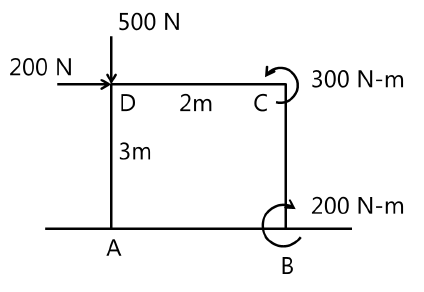5 M
1(b) Find forces in members AB, AE, ED, BC, CD.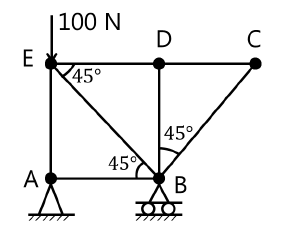x" />
5 M
1(c) Figure shows a plot of ax versus time for a particle moving along x-axis. What is the speed and distance covered by the particle after 50 seconds?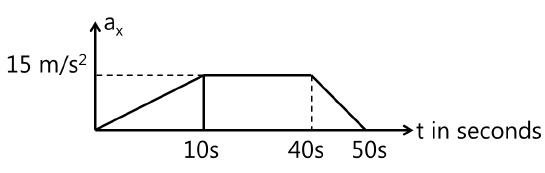5 M
1(d) By what percentage the range of projectile is increased if initial velocity is increased by 5%?
5 M
1(e) A ball is dropped from a height of 12m upon a horizontal slab. If it rebounds to height of 4m, find the co-efficient of restitution.
5 M

2(a) Three cylinders are piled up in a rectangular channel as shown in the figure. Determine the reactions at point 6 between the cylinder A and the vertical wall of the channel. (Cylinder A: radius = 4cm, m=15kg,
Cylinder B: radius = 6cm, m=40kg,
Cylinder C: radius = 5cm, m=20kg).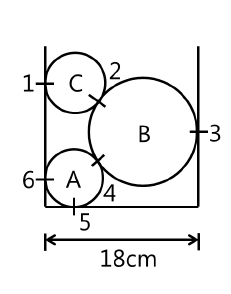12 M
2(b) Two identical balls of 120gm collide when they are moving with velocties as shown in figure. Determine the velocities of the balls A and B completely after the impact. Take e=0.8.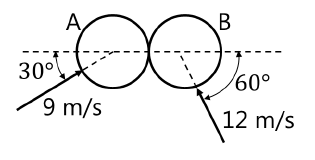8 M

3(a) A simply supported pin jointed truss is loaded and supported as shown in the following figure. Identify the members carrying zero force and find forces in members by method of joints.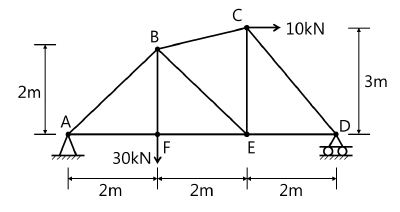12 M
3(b) A particle moves in a plane with constatnt acceleration a = 4i m/s2. At t = 0 the velocity of the particle was vo = i + 1.732j m/s. Find velocity of the particle at t = 1sec.
8 M

4(a) Determine the tension developed in cords attached to each block and the accelerations of the blocks when the system shown is released from rest. Neglect the mass of the pulleys and cords.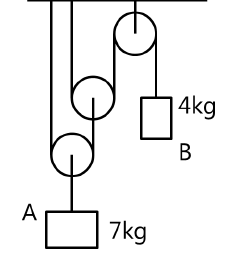12 M
4(b) The water sprinkler positioned at the base of a hill releases a stream of water with a velocity of 6 m/s as shown. Determine the point B(x,y) where the water particles strike the ground on the hill. Assume that the hill is defined the the equation y=0.2x2 m and neglect the size of the sprinkler.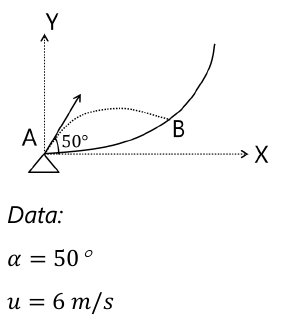8 M

5(a) Two blocks A (mass 10 kg), B (mass 28 kg) are separated by 12 m as shown in the figure. If the bloks start moving, find the time t when the blocks collide. Assume ? = 0.25 for block A and plane and ? = 0.10 for block B ad plane.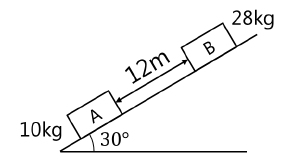12 M
5(b) Find the reactions at the supports of the beam applying conditions of equilibrium.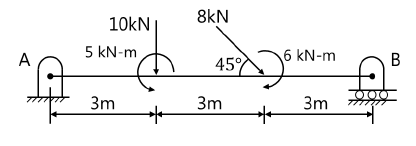8 M

6(a) A particle moves along a circle of radius 20 cm so that s=20 ?t2 cm. Find its tangential and normal acceleration after it has completed a revolution.
8 M
6(b) Find the centroid of the area shown in figure. Also find the moment of inertia of the same area about x axis.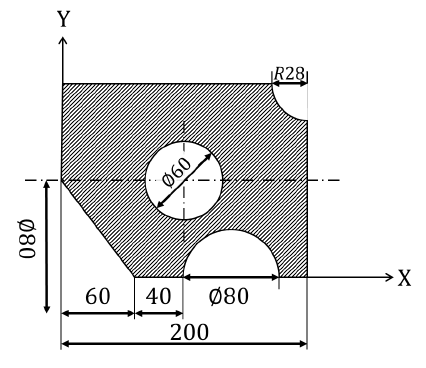12 M

7(a) In the mechanism shown, find velocity of point C and angular velocity of link BC if angular velocity of link AB is 4 rad/sec. Solve the problem when link AB and link BC make angle 450 with horizontal as shown in the figure.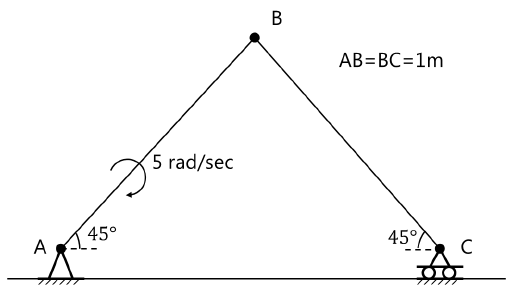8 M
7(b) A belt 120 mm wide and 8 mm thick is transmitting power at a belt speed of 1200 m/min. The angle of lap of the smaller pulley is 160° and coefficient of friction is 0.3. The maximum permissible stress in the belt is 2 N/mm2 and the mass of the belt is 0.8 kg/m. find the power transmitted ad the initial tension in the belt. Also find the maximum power that can be transmitted and the corresponding belt speed.
12 M

More question papers from Engineering Mechanics